﻿ C : Insert a new node at the end of a Singly Linked List

# C Exercises: Insert a new node at the end of a Singly Linked List

## C Singly Linked List : Exercise-5 with Solution

Write a program in C to insert a new node at the end of a Singly Linked List.

Pictorial Presentation: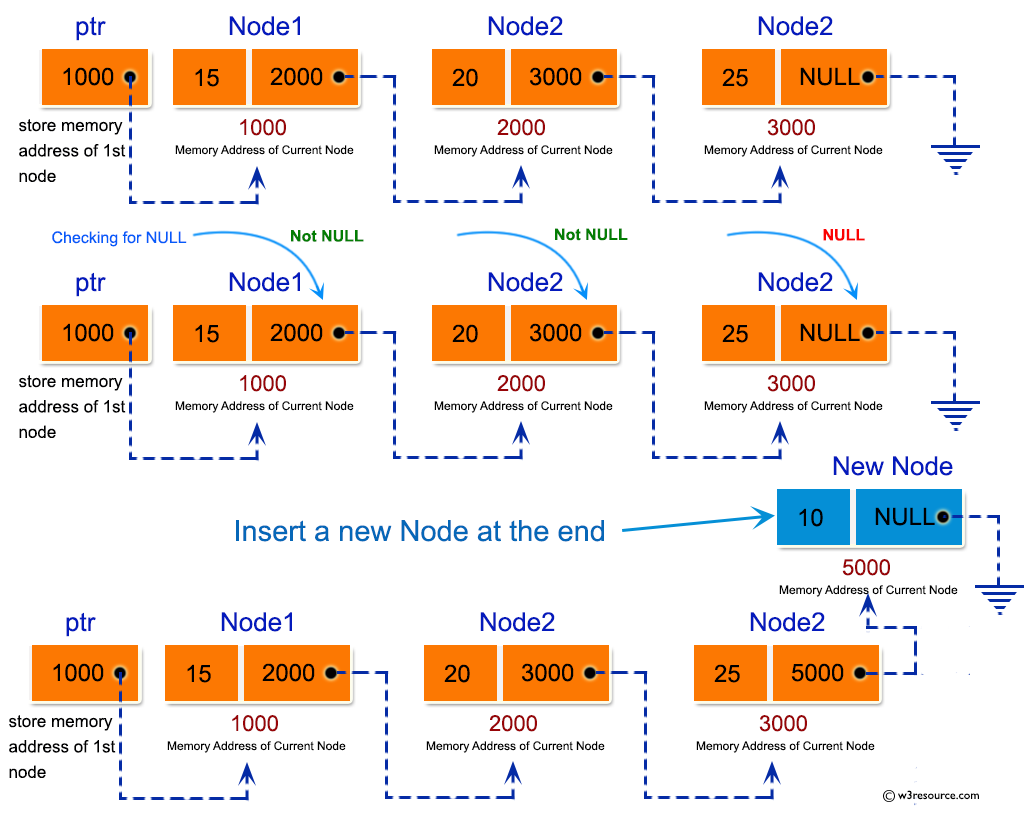Sample Solution:

C Code:

``````#include <stdio.h>
#include <stdlib.h>

struct node
{
int num;                    //Data of the node
struct node *nextptr;       //Address of the node
}*stnode;

void createNodeList(int n);     //function to create the list
void NodeInsertatEnd(int num);	//function to insert node at the end
void displayList();             //function to display the list

int main()
{
int n,num;
printf("\n\n Linked List : Insert a new node at the end of a Singly Linked List :\n");
printf("-------------------------------------------------------------------------\n");

printf(" Input the number of nodes : ");
scanf("%d", &n);
createNodeList(n);
printf("\n Data entered in the list are : \n");
displayList();
printf("\n Input data to insert at the end of the list : ");
scanf("%d", &num);
NodeInsertatEnd(num);
printf("\n Data, after inserted in the list are : \n");
displayList();
return 0;
}
void createNodeList(int n)
{
struct node *fnNode, *tmp;
int num, i;
stnode = (struct node *)malloc(sizeof(struct node));
if(stnode == NULL) //check whether the stnode is NULL and if so no memory allocation
{
printf(" Memory can not be allocated.");
}
else
{
// reads data for the node through keyboard
printf(" Input data for node 1 : ");
scanf("%d", &num);

stnode-> num = num;
tmp = stnode;
for(i=2; i<=n; i++)
{
fnNode = (struct node *)malloc(sizeof(struct node));
if(fnNode == NULL) //check whether the fnnode is NULL and if so no memory allocation
{
printf(" Memory can not be allocated.");
break;
}
else
{
printf(" Input data for node %d : ", i);
scanf(" %d", &num);
fnNode->num = num;      // links the num field of fnNode with num
fnNode->nextptr = NULL; // links the address field of fnNode with NULL
tmp->nextptr = fnNode; // links previous node i.e. tmp to the fnNode
tmp = tmp->nextptr;
}
}
}
}

void NodeInsertatEnd(int num)
{
struct node *fnNode, *tmp;
fnNode = (struct node*)malloc(sizeof(struct node));
if(fnNode == NULL)
{
printf(" Memory can not be allocated.");
}
else
{
fnNode->num = num;     //Links the data part
fnNode->nextptr = NULL;
tmp = stnode;
while(tmp->nextptr != NULL)
tmp = tmp->nextptr;
}
}

void displayList()
{
struct node *tmp;
if(stnode == NULL)
{
printf(" No data found in the empty list.");
}
else
{
tmp = stnode;
while(tmp != NULL)
{
printf(" Data = %d\n", tmp->num);   // prints the data of current node
tmp = tmp->nextptr;                 // advances the position of current node
}
}
}
```
```

Sample Output:

```Linked List : Insert a new node at the end of a Singly Linked List :
-------------------------------------------------------------------------
Input the number of nodes : 3
Input data for node 1 : 5
Input data for node 2 : 6
Input data for node 3 : 7

Data entered in the list are :
Data = 5
Data = 6
Data = 7

Input data to insert at the end of the list : 8

Data, after inserted in the list are :
Data = 5
Data = 6
Data = 7
Data = 8
```

Flowchart: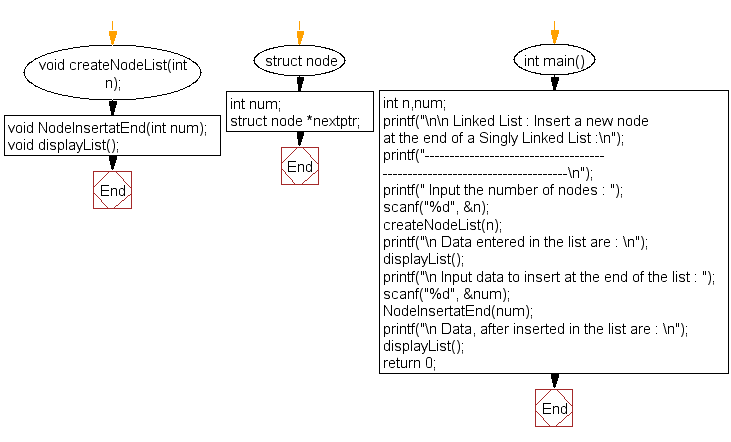createNodeList() :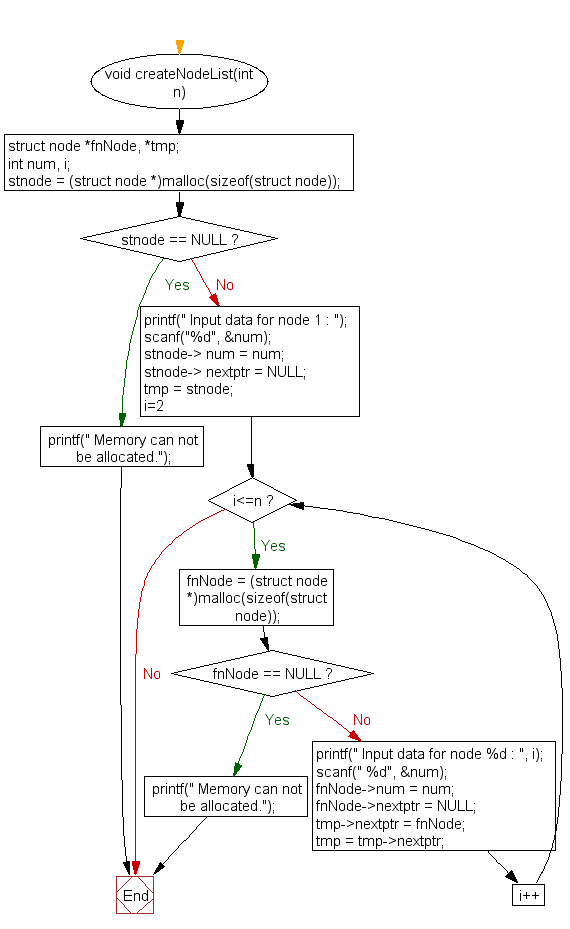NodeInsertatEnd() :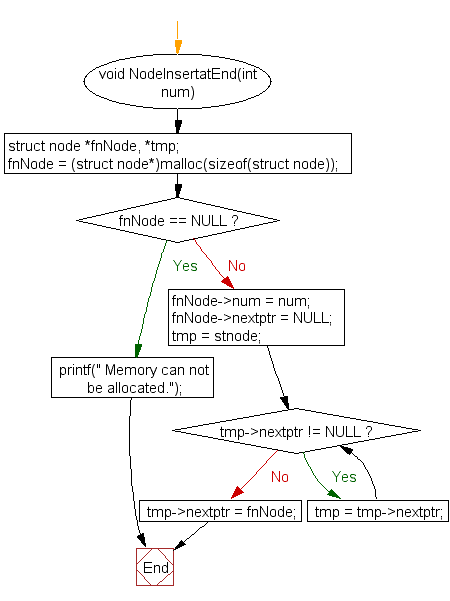displayList() :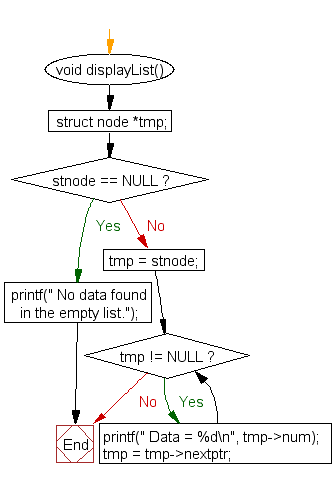C Programming Code Editor:

What is the difficulty level of this exercise?

Test your Programming skills with w3resource's quiz.

﻿